# Texas Go Math Grade 6 Module 17 Answer Key Analyzing and Comparing Data

Refer to our Texas Go Math Grade 6 Answer Key Pdf to score good marks in the exams. Test yourself by practicing the problems from Texas Go Math Grade 6 Module 17 Answer Key Analyzing and Comparing Data.

## Texas Go Math Grade 6 Module 17 Answer Key Analyzing and Comparing Data

Essential Question
How can you use solve real-world problems by displaying, analyzing, and summarizing data?Complete these exercises to review skills you will need for this chapter.

Find the quotient.

Question 1.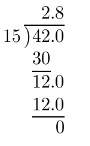Include a decimal point and zero on the dividend until there is no remainder.

The quotient is 2.8.

Question 2.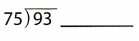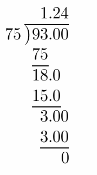Include a decimal point and zero on the dividend until there is no remainder.
The quotient is 1.24.

Question 3.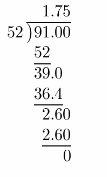Include a decimal point and zero on the dividend until there is no remainder.

The quotient is 1.75.

Question 4.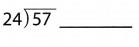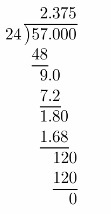Include a decimal point and zero on the dividend until there is no remainder.

The quotient is 2.375.

Question 5.
How many goals did Dion score? ________________
How many goals did Dion score? 3 goals

Study the given bar graph to identify the height on Dion’s graph. It is 3. This implies that Dion scored 3 goals.

Dion scored 3 goals

Question 6.
Which two players together scored the same number of goals as Jeff? _______
Study the given bar graph to identify the height on Jeffs graph. It is 4. This implies that Jeff scored 4 goals. This implies that the total number of goals scored is 4, therefore, it can be seen that Dion and Ted together scored 4 goals

Dion and Ted together scored as many goals as Jeff.

Question 7.
How many fewer goals than Cesar did Alec score? __________
Ceaser scored 6 goals white Alec scored 5, so Ceaser scored 6 – 5 = 1 more goal than Alec.

Ceaser scored 1 more goal than Alec

Visualize Vocabulary

Use the review words to complete the chart.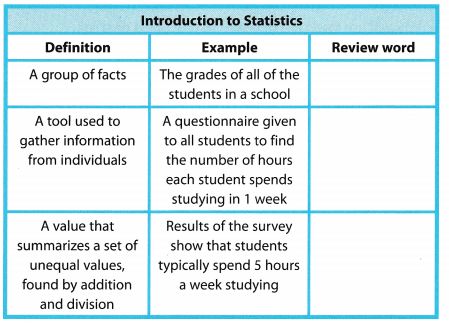Understand Vocabulary

Complete the sentences using the preview words.

Question 1.
The average of a data set is the ___.
The average of a data set is the mean.

The missing word that will complete the sentence is mean.

Question 2.
The ____________________ is the middle value of a data set.